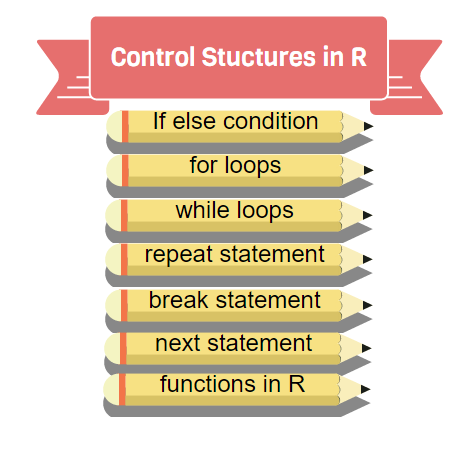We will learn about control structures loops used in R. Control strcutures in R contains conditionals, loop statements like any other programming languages.Loops are very important and forms backbone to any programming languages.

## If else statement:

#See the code syntax below for if else statement
x=10
if(x>1){
print("x is greater than 1")
}else{
print("x is less than 1")
} 
##  "x is greater than 1"
#See the code below for nested if else statement
x=10
if(x>1 & x<7){
print("x is between 1 and 7")}else if(x>8 & x< 15){
print("x is between 8 and 15")
}
##  "x is between 8 and 15"

## For loops:

As we know for loops are used for iterating items

 #Below code shows for  loop implementation
x = c(1,2,3,4,5)
for(i in 1:5){
print(x[i])
}
##  1
##  2
##  3
##  4
##  5

## While loop:

#Below code shows while loop in R
x = 2.987
while(x <= 4.987) {
x = x + 0.987
print(c(x,x-2,x-1))
}
##  3.974 1.974 2.974
##  4.961 2.961 3.961
##  5.948 3.948 4.948

## Repeat Loop

The repeat loop is an infinite loop and used in association with a break statement.

#Below code shows repeat loop:
a = 1
repeat{
print(a)
a = a+1
if(a > 4)break}
##  1
##  2
##  3
##  4

## Break statement:

A break statement is used in a loop to stop the iterations and flow the control outside of the loop.

 #Below code shows break statement:
x = 1:10
for (i in x){
if (i == 2){
break
}
print(i)
}
##  1

## Next statement:

Next statement enables to skip the current iteration of a loop without terminating it.

 #Below code shows next statement
x = 1: 4
for (i in x) {
if (i == 2){
next}
print(i)
}
##  1
##  3
##  4